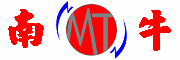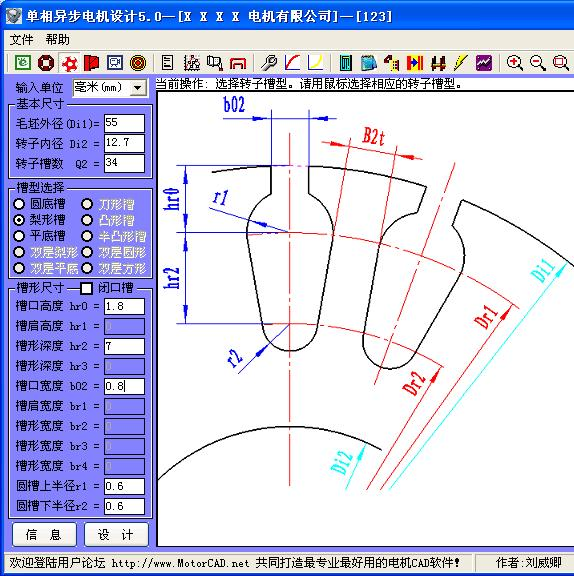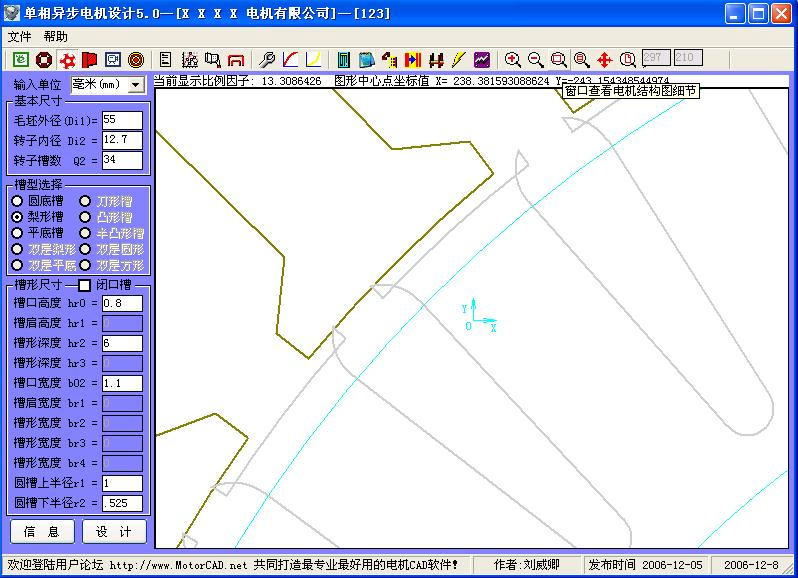# 南牛电机设计软件――专业智能自动设计软件，人人都能设计电机！# [推荐]新手入门教程(图片较多,请慢慢打开)

2006-12-8 19:54:00 来源:南牛公司原创 作者:管理员 点击：10748

毛坯外径：5.5厘米(cm)

转子内径：1.27厘米(cm)

转子槽数：34

转子槽形：梨形槽

hr0=.08厘米(cm)----->此数据输入是错误的,应该为0.08+0.1=0.18cm=1.8mm

hr1=0厘米(cm)          请仔细观察输入数据时的图片提示,注意保证其几何关系

hr2=.06厘米(cm)

hr3=0厘米(cm)

b02=.11厘米(cm)----->此数据也和你提供的数据不符合

br1=0厘米(cm)

br2=0厘米(cm)

br3=0厘米(cm)

br4=0厘米(cm)

r1=.1厘米(cm)----->此数据也和你提供的数据不符合

r2=.525厘米(cm)----->此数据也和你提供的数据不符合